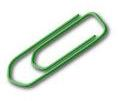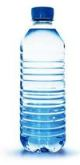# Weight and Mass

Weight is the measure of how heavy an object is. Weight is measured in standard customary units.

The mass of an object is the amount of material it contains. Mass is measured in standard metric units.

For everyday purposes, when you're on the surface of the earth, the difference is not important. But if you measure something on another planet, its mass will be the same as it is on earth—but its weight will be different. (Weight depends on gravity, and gravity is different on other planets! This is why when you're floating in space, you're weightless. You still have mass, though,)

## Metric Units

The gram and kilogram are two units used to measure mass in the metric system .

From the gram, we get the rest of the metric units using the standard metric prefixes.

 Milligram(mg) $0.001$ gram or $\frac{1}{1000}$ gram Centigram(cg) $0.01$ gram or $\frac{1}{100}$ gram Decigram (dg) $0.1$ gram or $\frac{1}{10}$ gram Gram(g) $1,000$ milligrams Dekagram (dag) $10$ grams Hectogram (hg) $100$ grams Kilogram (kg) $1,000$ grams Metric ton(t) $1,000$ kilograms

A paper clip has a mass of about $1$ gram.A $1$ liter bottle of water has a mass of $1$ kilogram (plus a little bit extra for the plastic).## U.S. Customary Units

The system for measuring weight (not mass) in the customary system is based on ounce, pound, and ton. The basic unit of weight is a pound(lb).

$\overline{)\begin{array}{|l|}\hline 1\text{\hspace{0.17em}}\text{\hspace{0.17em}}\text{pound}\text{\hspace{0.17em}}\left(\text{lb}\right)\text{\hspace{0.17em}}=\text{\hspace{0.17em}}16\text{\hspace{0.17em}}\text{\hspace{0.17em}}\text{ounces}\text{\hspace{0.17em}}\left(\text{oz}\right)\\ 1\text{\hspace{0.17em}}\text{\hspace{0.17em}}\text{ton}\text{\hspace{0.17em}}\left(\text{T}\right)\text{\hspace{0.17em}}\text{\hspace{0.17em}}\text{\hspace{0.17em}}\text{\hspace{0.17em}}\text{\hspace{0.17em}}\text{\hspace{0.17em}}\text{\hspace{0.17em}}\text{\hspace{0.17em}}=\text{\hspace{0.17em}}2,000\text{\hspace{0.17em}}\text{\hspace{0.17em}}\text{pounds}\\ \hline\end{array}}$

An ounce is the smallest unit of weight. A strawberry has a weight of about $1$ ounce.A car has a weight of about $2$ tons.To convert from one unit to another, we can use unit analysis . For example, a truck weights $6,000$ pounds. How many tons is this?

We know that $1\text{\hspace{0.17em}}\text{\hspace{0.17em}}\text{ton}=2,000\text{\hspace{0.17em}}\text{\hspace{0.17em}}\text{pounds}$ .

To convert pounds to tons, you can divide $6,000$ by $2,000$ .

$6,000÷2,000=3\text{\hspace{0.17em}}\text{\hspace{0.17em}}\text{tons}$ .

Note:

(a) To convert from larger units to smaller units, multiply by the appropriate unit ratio.

(b) To convert from smaller units to larger units, multiply by the reciprocal of the appropriate unit ratio.

(c) Multiplying or dividing by a unit ratio is mathematically equivalent to using a proportion to convert between units of measure .